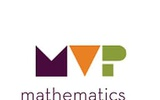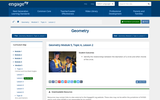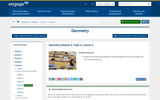Updating search results...

# 29 Results

View
Selected filters:
• NC.M3.G-C.2Conditional Remix & Share Permitted
CC BY-NC-SA
Rating
0.0 stars
Overview:

This is a unit length multi-learning style project that teaches the theorems and properties of Circles for the NC Math 3 curriculum. It includes hands on manipulatives using Exploragons, Digital Explorations in Geogebra, and a differentiating set of homework exercises through CK12.org.

Subject:
Mathematics
Material Type:
Activity/Lab
Author:
William Allred
Carrie Robledo
05/19/2021Conditional Remix & Share Permitted
CC BY-NC-SA
Rating
0.0 stars
Overview:

In this task students consider the similarity of circles by examining two different transformation strategies that map one circle onto another. In the first strategy one circle is translated so that the center of the circles coincide. The inner circle can then be enlarged to carry it onto the outer circle, or the outer circle can be shrunk to carry it onto the inner circle. Students are asked to determine the scale factors for both the enlargement and the reduction. In the second strategy students observe that any circle can be mapped onto any other circle by dilation. Students are also asked to find the scale factor of this dilation, which is the same scale factor as the enlargement (or reduction) factor used in the first strategy. Students are also given the opportunity to draw similar figures inscribed within the two circles, which has the potential of surfacing some observations about central and inscribed angles, and the relationship between tangent lines and radii.

Subject:
Math 3
Material Type:
Lesson
Author:
The Mathematics Vision Project
03/12/2020Rating
0.0 stars
Overview:

This resource is a unit about circles. Lessons and practice on definitions and application of definitions and theorems.

Subject:
Mathematics
Material Type:
Unit of Study
Provider:
Delaware Department of Education
06/07/2018Conditional Remix & Share Permitted
CC BY-NC-SA
Rating
0.0 stars
Overview:

The purpose of this task is to review and practice theorems and formulas associated with circles. Students will also draw upon ideas of similarity as well as right triangle trigonometry relationships to find the lengths of line segments. 30°-60°-90° triangles appear frequently in this task, so there is an opportunity to emphasize the relationships between the sides and how one can find the exact values of the lengths of the sides in this special right triangle.

Subject:
Math 3
Material Type:
Lesson
Author:
The Mathematics Vision Project
03/12/2020Conditional Remix & Share Permitted
CC BY-NC-SA
Rating
0.0 stars
Overview:

In the context of constructing circumscribed and inscribed circles for a triangle, students make observations about the relationships between central angles, inscribed angles and circumscribed angles. These observations are used to prove that angles inscribed in a semicircle are right angles, opposite angles of inscribed quadrilaterals are supplementary, and a tangent line to a circle is perpendicular to the radius drawn to the point of tangency.

Subject:
Math 3
Material Type:
Lesson
Author:
The Mathematics Vision Project
03/12/2020Conditional Remix & Share Permitted
CC BY-NC-SA
Rating
0.0 stars
Overview:

Using observations from a pushing puzzle, explore the converse of Thales' theorem: If triangle ABC is a right triangle, then A, B, and C are three distinct points on a circle with segment AC a diameter.
Prove the statement of Thales' theorem: If A, B, and C are three different points on a circle with segment AC a diameter, then angle ABC is a right angle.

Subject:
Math 1
Material Type:
Lesson
Author:
EngageNY
02/21/2020Conditional Remix & Share Permitted
CC BY-NC-SA
Rating
0.0 stars
Overview:

Identify the relationships between the diameters of a circle and other chords of the circle.

Subject:
Math 1
Material Type:
Lesson
Author:
EngageNY
02/21/2020Conditional Remix & Share Permitted
CC BY-NC-SA
Rating
0.0 stars
Overview:

Inscribe a rectangle in a circle.
Understand the symmetries of inscribed rectangles across a diameter.

Subject:
Math 3
Material Type:
Lesson
Author:
EngageNY
02/21/2020Conditional Remix & Share Permitted
CC BY-NC-SA
Rating
0.0 stars
Overview:

Explore the relationship between inscribed angles and central angles and their intercepted arcs.

Subject:
Math 3
Material Type:
Lesson
Author:
EngageNY
02/22/2020Conditional Remix & Share Permitted
CC BY-NC-SA
Rating
0.0 stars
Overview:

Prove the inscribed angle theorem: The measure of a central angle is twice the measure of any inscribed angle that intercepts the same arc as the central angle.
Recognize and use different cases of the inscribed angle theorem embedded in diagrams. This includes recognizing and using the result that inscribed angles that intersect the same arc are equal in measure.

Subject:
Math 3
Material Type:
Lesson
Author:
EngageNY
02/22/2020Conditional Remix & Share Permitted
CC BY-NC-SA
Rating
0.0 stars
Overview:

Define the angle measure of arcs, and understand that arcs of equal angle measure are similar.
Restate and understand the inscribed angle theorem in terms of arcs: The measure of an inscribed angle is half the angle measure of its intercepted arc.
Explain and understand that all circles are similar.

Subject:
Math 3
Material Type:
Lesson
Author:
EngageNY
02/22/2020Conditional Remix & Share Permitted
CC BY-NC-SA
Rating
0.0 stars
Overview:

Congruent chords have congruent arcs, and the converse is true.
Arcs between parallel chords are congruent.

Subject:
Math 3
Material Type:
Lesson
Author:
EngageNY
02/22/2020Conditional Remix & Share Permitted
CC BY-NC-SA
Rating
0.0 stars
Overview:

Students discover that a line is tangent to a circle at a given point if it is perpendicular to the radius drawn to that point.
Students construct tangents to a circle through a given point.
Students prove that tangent segments from the same point are equal in length.

Subject:
Math 3
Material Type:
Lesson
Author:
EngageNY
02/22/2020Conditional Remix & Share Permitted
CC BY-NC-SA
Rating
0.0 stars
Overview:

Students use tangent segments and radii of circles to conjecture and prove geometric statements, especially those that rely on the congruency of tangent segments to a circle from a given point.
Students recognize and use the fact if a circle is tangent to both rays of an angle, then its center lies on the angle bisector.

Subject:
Math 3
Material Type:
Lesson
Author:
EngageNY
02/22/2020Conditional Remix & Share Permitted
CC BY-NC-SA
Rating
0.0 stars
Overview:

Students use the inscribed angle theorem to prove other theorems in its family (different angle and arc configurations and an arc intercepted by an angle at least one of whose rays is tangent).
Students solve a variety of missing angle problems using the inscribed angle theorem.

Subject:
Math 3
Material Type:
Lesson
Author:
EngageNY
02/22/2020Conditional Remix & Share Permitted
CC BY-NC-SA
Rating
0.0 stars
Overview:

Students understand that an angle whose vertex lies in the interior of a circle intersects the circle in two points and that the edges of the angles are contained within two secant lines of the circle.
Students discover that the measure of an angle whose vertex lies in the interior of a circle is equal to half the sum of the angle measures of the arcs intercepted by it and its vertical angle.

Subject:
Math 3
Material Type:
Lesson
Author:
EngageNY
02/22/2020Conditional Remix & Share Permitted
CC BY-NC-SA
Rating
0.0 stars
Overview:

Students find the measures of angle/arcs and chords in figures that include two secant lines meeting outside a circle, where the measures must be inferred from other data.

Subject:
Math 3
Material Type:
Lesson
Author:
EngageNY
02/22/2020Conditional Remix & Share Permitted
CC BY-NC-SA
Rating
0.0 stars
Overview:

Students find “missing lengths” in circle-secant or circle-secant-tangent diagrams.

Subject:
Math 3
Material Type:
Lesson
Author:
EngageNY
02/22/2020Conditional Remix & Share Permitted
CC BY-NC-SA
Rating
0.0 stars
Overview:

Students show that a quadrilateral is cyclic if and only if its opposite angles are supplementary.
Students derive and apply the area of cyclic quadrilateral ABCD as 1/2 AB·CD·sin(w) where w is the measure of the acute angle formed by diagonals AB and CD.

Subject:
Math 3
Material Type:
Lesson
Author:
EngageNY
02/22/2020Conditional Remix & Share Permitted
CC BY-NC-SA
Rating
0.0 stars
Overview:

Students determine the area of a cyclic quadrilateral as a function of its side lengths and the acute angle formed by its diagonals.
Students prove Ptolemy’s theorem, which states that for a cyclic quadrilateral ABCD, AC·BD = AB·CD + BC·AD. They explore applications of the result.

Subject:
Math 3
Material Type:
Lesson
Author:
EngageNY